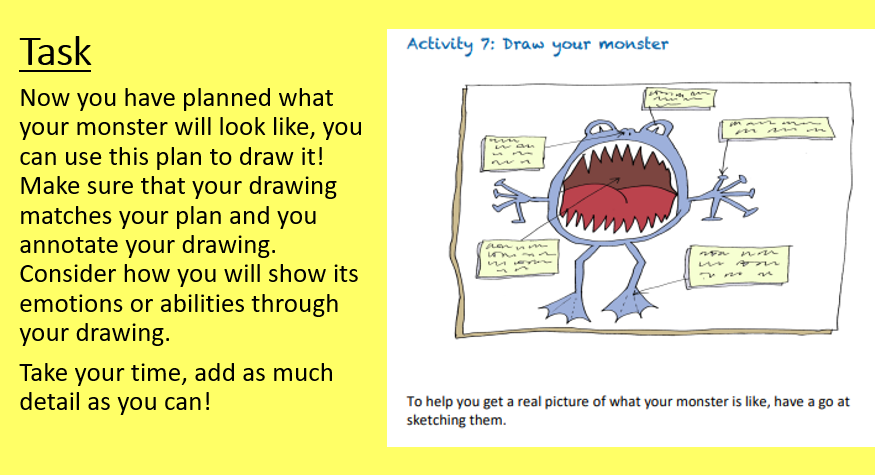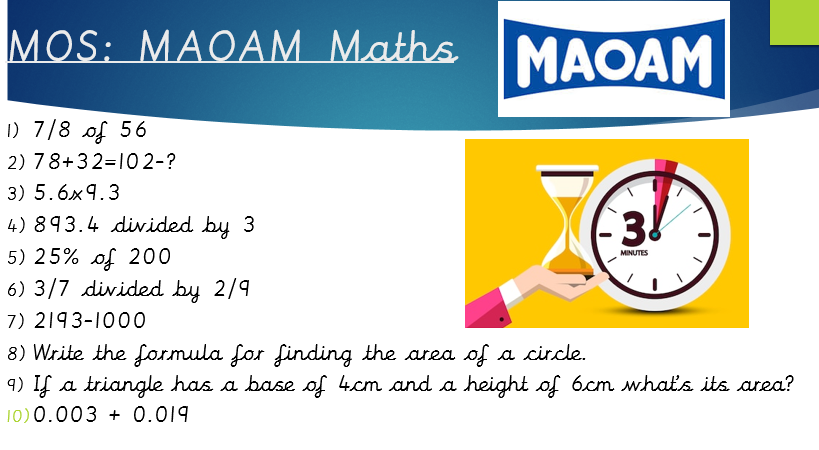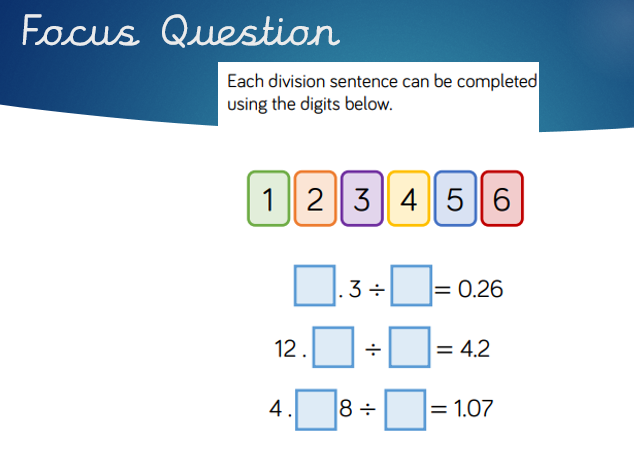# Daily Learning 08.07.2020Good morning everyone!  Here is a riddle to get your brains warmed up: what room has no windows or doors?  The answer is at the bottom of the blog.  Work through today's learning before reading the answer!

Mrs. Rowland Hill and Mrs. Manning wanted to share their bubble with you all as it is the last time they will all be together.  Looking at it now, we will miss every single one of you:Here is your English for today:We would love to see your pictures on Padlet or email them to your class teacher to scare them!

Here is your Maths for today:

Let's review the final 5 questions for Maoam Maths:6) Dividing fractions: Keep it, change it, flip it! 3/7 ÷ 2/9 is the same as 3/7 x 9/2.  Then we need to follow the method for multiplying fractions: multiply the numerators then multiply the denominators: 3 x 9 = 27 and 7 x 2 = 14, 27/14 or 1 13/14

7) The column method is unnecessary here as it is only the thousands column that is changing: 2193 - 1000 = 1193

8) The area of a circle is found by using the following formula: Area = π r 2, which is Pi (3.14) x the radius of the circle x the radius of the circle.  If the radius of the circle is 4cm, the area is calculated by 3.14 x 4 x 4 = 50.24cm2

9) The area of a triangle is half the base x the height, which is 2 x 6 = 12cm2

10) The column method works well here although this calculation can also be worked out mentally: 0.022